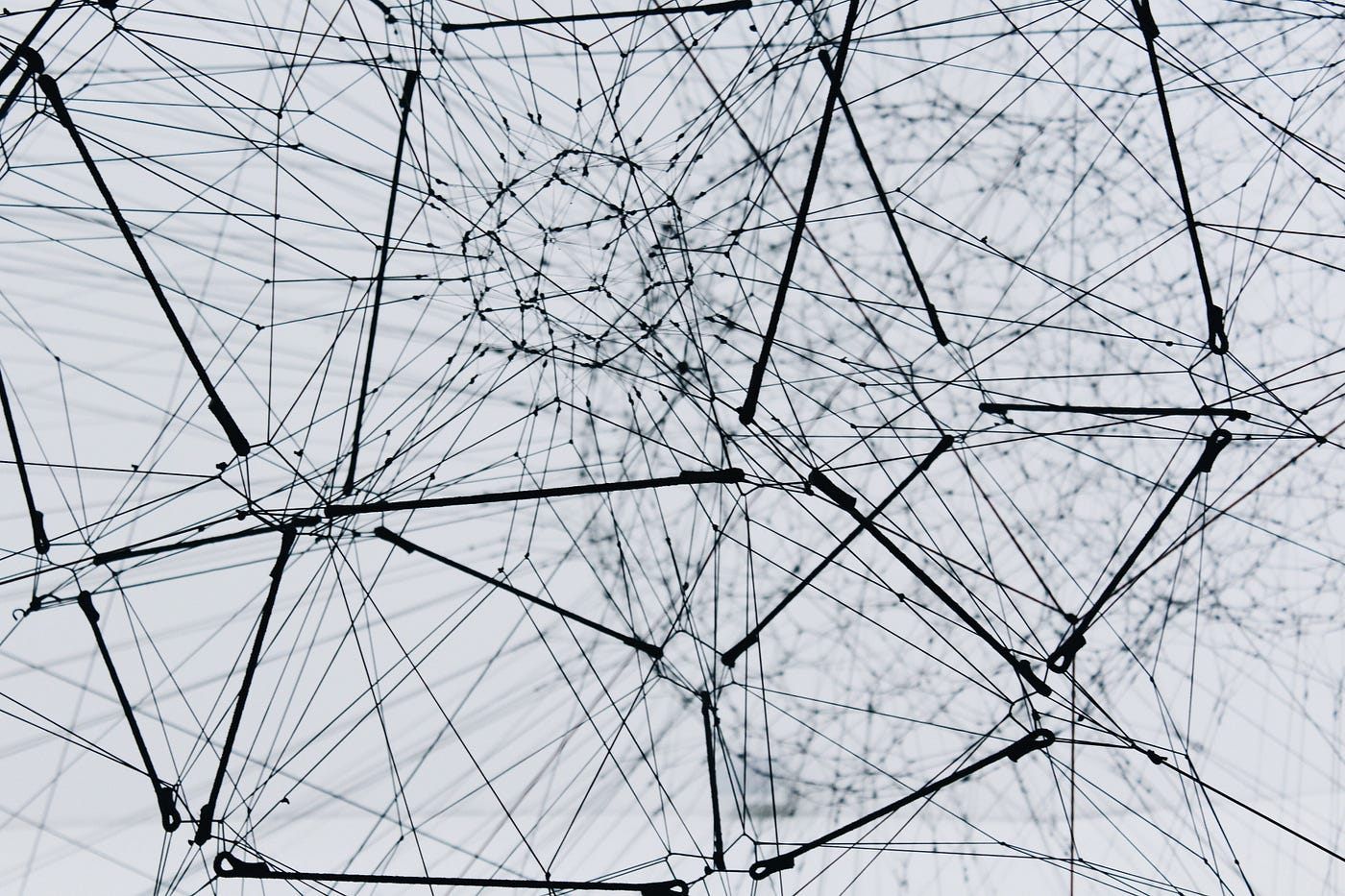# Residual Networks

In this post, we will learn about residual networks, why we need them, and …Photo by Alina Grubnyak on Unsplash

# Why We need Residuals?

Network depth has crucial importance, But Is learning better networks as easy as stacking more layers?
This was answered in Deep Residual Learning for Image Recognition paper when you try to add more layers and go deeper in your network, after a point, you face a problem that is named degradation: with the network depth increasing, accuracy gets saturated (which might be unsurprising) and then degrades rapidly. Unexpectedly, such degradation is not caused by overfitting, and adding more layers to a suitably deep model lead to higher training error

# What is Residual Connection?

Let us consider H(x) as an underlying mapping to be fit by a few stacked layers (not necessarily the entire net), with x denoting the inputs to the first of these layers. If one hypothesizes that multiple nonlinear layers can asymptotically approximate complicated functions2, then it is equivalent to hypothesize that they can asymptotically approximate the residual functions, i.e., H(x) — x (assuming that the input and output are of the same dimensions). So rather than expect stacked layers to approximate H(x), we explicitly let these layers approximate a residual function F(x):= H(x) — x. The original function thus becomes F(x)+x. Although both forms should be able to asymptotically approximate the desired functions (as hypothesized), the ease of learning might be different.

we consider a building block defined as:

## y = F(x, {Wi}) + x

Here x and y are the input and output vectors of the layers considered. The function F(x, {Wi}) represents the residual mapping to be learned.

# Is Vanishing our Main Problem?

We argue that this optimization difficulty is unlikely to be caused by vanishing gradients. These plain networks are trained with BN, which ensures forward propagated signals to have non-zero variances. We also verify that the backward propagated gradients exhibit healthy norms with BN. So neither forward nor backward signals vanish.

# But Why Residuals Work?

If the added layers can be constructed as identity mappings, a deeper model should have a training error no greater than its shallower counterpart. The degradation problem suggests that the solvers might have difficulties in approximating identity mappings by multiple nonlinear layers. With the residual learning reformulation, if identity mappings are optimal, the solvers may simply drive the weights of the multiple nonlinear layers toward zero to approach identity mappings.
In real cases, it is unlikely that identity mappings are optimal, but our reformulation may help to precondition the problem. If the optimal function is closer to an identity mapping than to a zero mapping, it should be easier for the solver to find the perturbations with reference to an identity mapping, than to learn the function as a new one.

# Different Kinds of Residual Blocks

There are two kinds of residual blocks :

## a)identical residual Blocks:

In an identical residual block, the output of the shortcut path and the main path are of the same dimensions. This is achieved by padding the input to each convolutional layer in the main path in such a way that the output and input dimensions remain the same.

b) Convolutional residual block

In this type of residual block, the skip-connection consists of a convolutional layer to resize the output of the shortcut path to be of the same dimension as that of the main path. The layer can also make use of different filter sizes, including 1×1, padding, and strides to control the dimension of the output volume.

References:

 He, K., Zhang, X., Ren, S. and Sun, J., 2016. Deep residual learning for image recognition. In Proceedings of the IEEE conference on computer vision and pattern recognition (pp. 770–778).

https://towardsdatascience.com/resnets-residual-blocks-deep-residual-learning-a231a0ee73d2

--

--